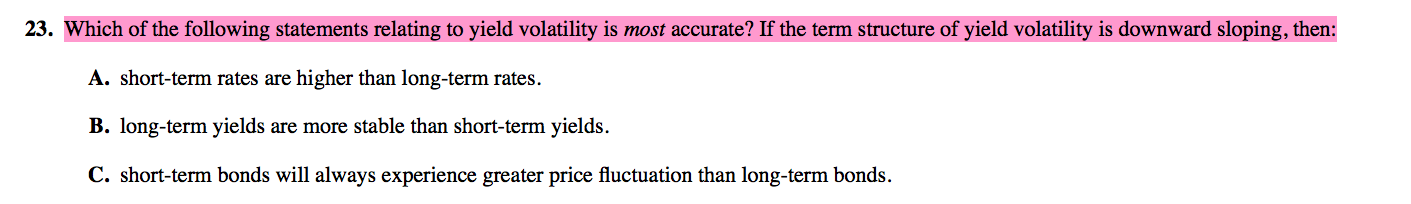CFA CFA Level 1 Effective duration for option bond

# Effective duration for option bond

• Author
Posts
•wannabe1988
Participant
• CFA Level 1
•36

Can someone help to explain why option bonds have lower effective duration compared to straight bond? I don’t really understand why changes in yield has lower effect on the price of an option bond. Is it due to option bonds have a more convex line than what is assumed by the effective duration estimation? At both higher price and lower price, due to the convexity, the rate of change in the relationship between price and yield are smaller than what is captured by the effective duration?

•Sophie Macon
Keymaster
•5

Hi @wannabe1988, good question, let me give this a go and see if it aids your understanding. @googs1484 and you are on the right track but I thought I’d break your discussion down to more basic level.

First, a couple of definitions:

1. Effective duration measures the % change in a bond’s price for a 1% change in yield. Note that effective duration assumes that the relationship is linear (it’s not!) at all levels of yield.
2. Hence, here’s where convexity comes in, which measures the % change in bond’s effective duration to 1% change in its yield. In short, convexity measures the rate of change of duration due to a yield change.
3. Value of callable bond = value of straight bond – value of embedded call option
4. Value of putable bond = value of straight bond + value of embedded put option

The effective duration of a bond with embedded option <= a straight bond because:

a) For a callable bond:
– if interest rate is high relative to bond coupon, it is unlikely to be called (redeemed) by the bond issuer, and therefore behaves similarly to a straight bond and have similar effective duration.
– if interest rate is low relative to bond coupon, it is likely to be called by the bond issuer and therefore there is effectively a cap on the upside potential of the bond prices (when interest rates drop). This means that the effective duration of the callable bond at this level is less than a straight bond (i.e. the change in bond price of a callable bond is less sensitive to a change in interest rate when interest rate is lower relative to bond coupon).

b) same logic for a putable bond:
– if interest rate is low relative to bond coupon, it is unlikely to be put (sold) by the bond holder, and therefore behaves similarly to a straight bond and have similar effective duration.
– if interest rate is high relative to bond coupon, it is likely to be sold (put back) by the bond holder as bond price falls, there is effectively a floor on a putable bond price due to the put option (when interest rate drops) vs. a straight bond. Therefore effective duration of a putable bond is less than a straight bond.

Slightly long-winded, but hope this helps!

•wannabe1988
Participant
• CFA Level 1
•4

I agreed with you. I am pretty sure these are the reasons why effective duration is as such but I cannot put my head around how the capping is affecting the effective duration (formula wise). If the capping decreases YTM, shouldn’t the effective duration increases? if effective duration decreases that means YTM increases greater than under option-free bond?

•googs1484
Participant
• CFA Level 3
•4

That’s a good explanation for sure. Mine was pretty dry sorry

•wannabe1988
Participant
• CFA Level 1
•4

By the way, I am trying to avoid creating too many threads but i hope it’s okay that I ask another question related to bond here.

For the following question, if the yield curve is downward sloping, isn’t that implied that the short-term bond is more volatile thus requires greater interest rate to compensate for the volatility? But according to CFAI answer, for such a yield curve, volatile short-term bond does not necessarily implies higher interest rate than long-term bond but I thought volatility usually implies higher interest rate?•googs1484
Participant
• CFA Level 3
•2

I could be wrong here but I’m pretty sure embedded options put caps on convexity. Or limits the convexity of the curve (yield= x axis, price=y axis). A call option would limit convexity to a mimimun yield the call owner can purchase a bond for, or max price.  A put allows the owner to sell a bond a minimum price.

•wannabe1988
Participant
• CFA Level 1
•2

Thanks a lot! that makes a lot of sense! 🙂

No apology is needed, @googs1484
Thanks for helping too!
•wannabe1988
Participant
• CFA Level 1
•1

Thanks for replying. Actually the answer is B. This is EOC from CFAI textbook

B is correct. If the term structure of yield volatility is downward-sloping, then short-term bond yields-to-maturity have greater volatility than for long-term bonds. Therefore, long-term yields are more stable than short-term yields. Higher volatility in short-term rates does not necessarily mean that the level of short-term rates is higher than long-term rates. With a downward-sloping term structure of yield volatility, short-term bonds will not always experience greater price fluctuation than long-term bonds. The estimated percentage change in a bond price depends on the modified duration and convexity as well as on the yield-to-maturity change.

(Institute 287)

Institute, CFA. 2015 CFA Level I Volume 5 Equity and Fixed Income. Wiley Global Finance, 2014-07-14. VitalBook file.

• 0

the answer is A . I’m not entirely sure where you’re getting the relationship between volatility and interest rates. Sort of seems like you’re conflating option value there. If anything, if volatility increases investors will have greater uncertainty about long term bonds and will demand a slightly higher premium to compensate (interest rates will increase)